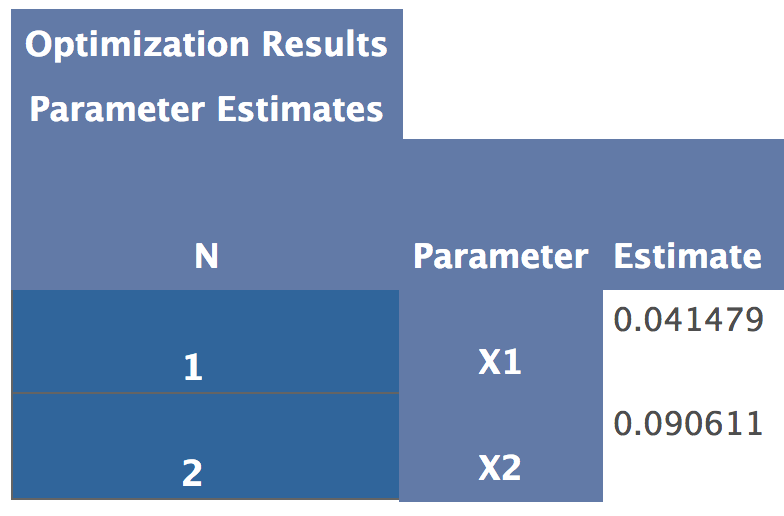## Assigning results of proc iml nlpqn optimization to macro variable.

Hi!

I currently have code which implements the nlpqn optimization method in proc iml. In my code I have an initial value x={val1 val2}. The code functions and produces output. I need to save/store the results of the procedure (x={newval1 newval2}) to a macro variable (or preferably two macro variables) to be used later in my code (outside of the proc iml procedure). I have tried

call symputx('macrovar1', x);

call symputx('macrovar2', x);

inside of the proc iml procedure which did not work (it saved the values of the original initial value instead of the new values for which the procedure solved). I have also tried

%let macrovar1 = x;

%let macrovar2 = x;

statements outside of the proc iml procedure which did not work either. Suggestions? Any advice would be greatly appreciated!

Thank you!

1 ACCEPTED SOLUTION

Accepted Solutions

## Re: Assigning results of proc iml nlpqn optimization to macro variable.

This example from the doc works fine for me:

``````%let result1=0;
%let result4=-1;

proc iml;
start F_HS43(x);
f = x*x` + x*x - 5*(x + x) - 21*x + 7*x;
return(f);
finish F_HS43;
start C_HS43(x);
c = j(3,1,0.);
c = 8 - x*x` - x + x - x + x;
c = 10 - x*x` - x*x - x*x + x + x;
c = 5 - 2.*x*x - x*x - x*x
- 2.*x + x + x;
return(c);
finish C_HS43;
x = j(1,4,1);
optn= j(1,11,.); optn= 0; optn= 3; optn=0;
call nlpqn(rc,xres,"F_HS43",x,optn) nlc="C_HS43";
print xres[L="Result"];

/* use SYMPUTX to create macro for a single numeric value */
call symputx("result1", xres);
call symputx("result2", xres);
call symputx("result3", xres);
call symputx("result4", xres);
quit;

%put &result1
&result2
&result3
&result4;

``````
7 REPLIES 7

## Re: Assigning results of proc iml nlpqn optimization to macro variable.

Update: I know why the %let statements outside of the proc iml don't work; stupid moment.

## Re: Assigning results of proc iml nlpqn optimization to macro variable.

It can be challeging to understand how macros work inside an interactive procedure. See the article "Macros and Loops in the SAS/IML language."

Using SYMPUTX should wok. I don't see anything wrong with the snippets you posted, but tha problem might be elsewhere in your program. Here is code that works. perhaps you can use it to find the problem. Notice that SYMPUTX converts a sclar numeric value into a character string and puts it in a macro variable (which is ALWAYS character!). You can use SYMPUT if you manually convert to a character string yourself.

``````proc iml;
/* use SYMPUTX to create macro for a single numeric value */
s = 1.234567;
call symputx("scalar", s);

/* use SYMPUT for character strings */
x = {1.23 3.456 7.8901};
call symput("vector", rowcat(char(x)));
quit;

%put scalar=&scalar;
%put vector=&vector;``````

## Re: Assigning results of proc iml nlpqn optimization to macro variable.

Hi Rick!

syputx does assign values to the macro variables but not the values solved for in the optimization. For example, my original initial value was x={0.0001 0.0901} and AFTER the optimization these parameters are x={.0414 0.08}. The problem is that the symputx is assigning the macro variables the values of the original inital value (0.0001 and 0.0901) instead of the new values (0.0414 and 0.08) as desired. I have placed the symputx calls at the end of the proc iml procedure (after I call nlpqn(rc,xres,"F_OPT",x,optn) with nlc="C_OPT").

I'll look through the documentation you sent, as well as continue to look online, but I figured I'd check with you as well.

Thanks!

## Re: Assigning results of proc iml nlpqn optimization to macro variable.

Create a simple example and post your code.   Unless I can reproduce the problem, I don't know what else to offer.

## Re: Assigning results of proc iml nlpqn optimization to macro variable.

That's fair. 🙂 Here's the outline of my code:

proc iml;

use dataset;

rea all var into VARS;

close dataset;

start F_OPT(x);   /*this would be my function which I'm looking to minimize*/

code for F_OPT here;

finish F_OPT;

start C_OPT(x);    /*this would be my (nonlinear) constraints*/

code for C_OPT here;

finish C_OPT;

x={initialval1 initialval2};

optn=j(1,11,.);

optn=4;

optn=3;

optn=0;

call nlpqn(rc,xres,"F_OPT",x,optn) nlc="C_OPT";

call symputx('macrovar1',x);    /*attempt at assigning result of nlpqn parameter to macrovar1*/

call symputx('macrovar2',x);    /*attempt at assigning result of nlpqn parameter to macrovar2*/

quit;

I hope this helps (and let me know if you need more)!

Thank you!

P.S. See attachments for output.## Re: Assigning results of proc iml nlpqn optimization to macro variable.

This example from the doc works fine for me:

``````%let result1=0;
%let result4=-1;

proc iml;
start F_HS43(x);
f = x*x` + x*x - 5*(x + x) - 21*x + 7*x;
return(f);
finish F_HS43;
start C_HS43(x);
c = j(3,1,0.);
c = 8 - x*x` - x + x - x + x;
c = 10 - x*x` - x*x - x*x + x + x;
c = 5 - 2.*x*x - x*x - x*x
- 2.*x + x + x;
return(c);
finish C_HS43;
x = j(1,4,1);
optn= j(1,11,.); optn= 0; optn= 3; optn=0;
call nlpqn(rc,xres,"F_HS43",x,optn) nlc="C_HS43";
print xres[L="Result"];

/* use SYMPUTX to create macro for a single numeric value */
call symputx("result1", xres);
call symputx("result2", xres);
call symputx("result3", xres);
call symputx("result4", xres);
quit;

%put &result1
&result2
&result3
&result4;

``````

## Re: Assigning results of proc iml nlpqn optimization to macro variable.

That did it! You're amazing! I just needed to use xres instead of x (which totally makes sense now that I see it)! Thank you! Thank you! Thank you!

From The DO Loop